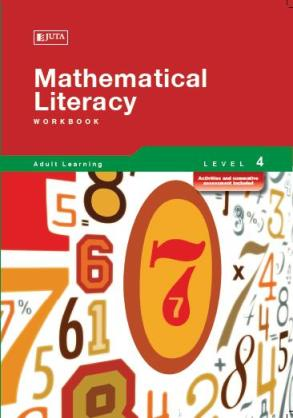## Mathematical Literacy 4

• R 120.00
• Save R 60

Mathematical Literacy Level 4 is divided into modules of which each module covers a unit standard. Specific outcomes for each unit standard are divided into well illustrated, interactive lessons that develop the skills of calculations, number knowledge, shapes and angles. A self assessment exercise is given at the end of each module.

We Also Recommend